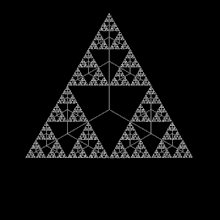### Fractals and Coding

This post is for Maths teachers who teach fractals and assumes knowledge of Fractal theory.

# Fractals and Recursion

Recursion is a recurring function that calls or refers to itself.
A function is a name given to a series of steps and is used to simplify programming by referring to or "calling" the function.https://guide.pencilcode.net/edit/functions/
For example the code below is to draw a square. The first part defines "square" as a function and tells the program what "square" means and then uses to the word "square" to draw multiple squares of different sizes and colours without the need to write all the code.

square = (size) -> for y in [1..4] fd size rt 90 pen red square 80 jump 15, 15 pen firebrick square 50https://en.wikipedia.org/wiki/Fractal
A Fractal is a curve or geometrical figure, each part of which has the same statistical character as the whole. They are useful in modelling structures (such as snowflakes) in which similar patterns recur at progressively smaller scales, and in describing partly random or chaotic phenomena such as crystal growth and galaxy formation.

Here are some examples of activities that could be used to illustrate fractals using a program called pencilcode.net

# 14. Recursion

spiral = (x) ->
if x > 0
fd x * 10
rt 90
spiral x - 1
lt 90
bk x * 10
pen red
spiral 10

speed 1000
fern = (x) ->
if x > 1
fd x
rt 95
fern x * .4
lt 190
fern x * .4
rt 100
fern x * .8
lt 5
bk x
pen green
fern 50

speed Infinity
flake = (x) ->
if x < 3 then fd x
else
flake x / 3
lt 60
flake x / 3
rt 120
flake x / 3
lt 60
flake x / 3
pen 'path'
for s in [1..3]
flake 150
rt 120
fill 'azure strokeStyle navy'

http://bit.ly/Fractals2018